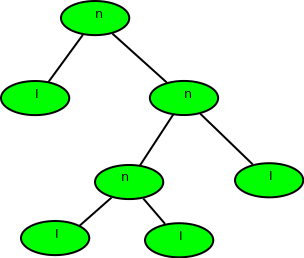# Calculate depth of a full Binary tree from Preorder

• Difficulty Level : Medium
• Last Updated : 20 May, 2021

Given preorder of a binary tree, calculate its depth(or height) [starting from depth 0]. The preorder is given as a string with two possible characters.

1. ‘l’ denotes the leaf
2. ‘n’ denotes internal node

The given tree can be seen as a full binary tree where every node has 0 or two children. The two children of a node can ‘n’ or ‘l’ or mix of both.
Examples :

Attention reader! Don’t stop learning now. Get hold of all the important DSA concepts with the DSA Self Paced Course at a student-friendly price and become industry ready.  To complete your preparation from learning a language to DS Algo and many more,  please refer Complete Interview Preparation Course.

In case you wish to attend live classes with experts, please refer DSA Live Classes for Working Professionals and Competitive Programming Live for Students.

```Input  : nlnll
Output : 2
Explanation :``````Input  : nlnnlll
Output : 3```` `

Preorder of the binary tree is given so traverse
Also, we would be given a string of char (formed of ‘n’ and ‘l’), so there is no need to implement tree also.
The recursion function would be:
1) Base Case: return 0; when tree[i] = ‘l’ or i >= strlen(tree)
2) find_depth( tree[i++] ) //left subtree
3) find_depth( tree[i++] ) //right subtree
Where i is the index of the string tree.

## C++

 `// C++ program to find height of full binary tree``// using preorder``#include ``using` `namespace` `std;` `// function to return max of left subtree height``// or right subtree height``int` `findDepthRec(``char` `tree[], ``int` `n, ``int``& index)``{``    ``if` `(index >= n || tree[index] == ``'l'``)``        ``return` `0;` `    ``// calc height of left subtree (In preorder``    ``// left subtree is processed before right)``    ``index++;``    ``int` `left = findDepthRec(tree, n, index);` `    ``// calc height of right subtree``    ``index++;``    ``int` `right = findDepthRec(tree, n, index);` `    ``return` `max(left, right) + 1;``}` `// Wrapper over findDepthRec()``int` `findDepth(``char` `tree[], ``int` `n)``{``    ``int` `index = 0;``    ``findDepthRec(tree, n, index);``}` `// Driver program``int` `main()``{``    ``// Your C++ Code``    ``char` `tree[] = ``"nlnnlll"``;``    ``int` `n = ``strlen``(tree);` `    ``cout << findDepth(tree, n) << endl;` `    ``return` `0;``}`

## Java

 `// Java program to find height``// of full binary tree using``// preorder``import` `java .io.*;` `class` `GFG``{``    ``// function to return max``    ``// of left subtree height``    ``// or right subtree height``    ``static` `int` `findDepthRec(String tree,``                            ``int` `n, ``int` `index)``    ``{``        ``if` `(index >= n ||``            ``tree.charAt(index) == ``'l'``)``            ``return` `0``;` `        ``// calc height of left subtree``        ``// (In preorder left subtree``        ``// is processed before right)``        ``index++;``        ``int` `left = findDepthRec(tree,``                                ``n, index);` `        ``// calc height of``        ``// right subtree``        ``index++;``        ``int` `right = findDepthRec(tree, n, index);` `        ``return` `Math.max(left, right) + ``1``;``    ``}` `    ``// Wrapper over findDepthRec()``    ``static` `int` `findDepth(String tree,``                         ``int` `n)``    ``{``        ``int` `index = ``0``;``        ``return` `(findDepthRec(tree,``                             ``n, index));``    ``}` `    ``// Driver Code``    ``static` `public` `void` `main(String[] args)``    ``{``        ``String tree = ``"nlnnlll"``;``        ``int` `n = tree.length();``        ``System.out.println(findDepth(tree, n));``    ``}``}` `// This code is contributed``// by anuj_67.`

## Python3

 `#Python program to find height of full binary tree``# using preorder``    ` `# function to return max of left subtree height``# or right subtree height``def` `findDepthRec(tree, n, index) :` `    ``if` `(index[``0``] >``=` `n ``or` `tree[index[``0``]] ``=``=` `'l'``):``        ``return` `0` `    ``# calc height of left subtree (In preorder``    ``# left subtree is processed before right)``    ``index[``0``] ``+``=` `1``    ``left ``=` `findDepthRec(tree, n, index)` `    ``# calc height of right subtree``    ``index[``0``] ``+``=` `1``    ``right ``=` `findDepthRec(tree, n, index)``    ``return` `(``max``(left, right) ``+` `1``)` `# Wrapper over findDepthRec()``def` `findDepth(tree, n) :` `    ``index ``=` `[``0``]``    ``return` `findDepthRec(tree, n, index)` `        ` `# Driver program to test above functions``if` `__name__ ``=``=` `'__main__'``:``    ``tree``=` `"nlnnlll"``    ``n ``=` `len``(tree)` `    ``print``(findDepth(tree, n))` `# This code is contributed by SHUBHAMSINGH10`

## C#

 `// C# program to find height of``// full binary tree using preorder``using` `System;` `class` `GFG {` `    ``// function to return max of left subtree``    ``// height or right subtree height``    ``static` `int` `findDepthRec(``char``[] tree, ``int` `n, ``int` `index)``    ``{``        ``if` `(index >= n || tree[index] == ``'l'``)``            ``return` `0;` `        ``// calc height of left subtree (In preorder``        ``// left subtree is processed before right)``        ``index++;``        ``int` `left = findDepthRec(tree, n, index);` `        ``// calc height of right subtree``        ``index++;``        ``int` `right = findDepthRec(tree, n, index);` `        ``return` `Math.Max(left, right) + 1;``    ``}` `    ``// Wrapper over findDepthRec()``    ``static` `int` `findDepth(``char``[] tree, ``int` `n)``    ``{``        ``int` `index = 0;``        ``return` `(findDepthRec(tree, n, index));``    ``}` `    ``// Driver program``    ``static` `public` `void` `Main()``    ``{``        ``char``[] tree = ``"nlnnlll"``.ToCharArray();``        ``int` `n = tree.Length;``        ``Console.WriteLine(findDepth(tree, n));``    ``}``}` `// This code is contributed by vt_m.`

## Javascript

 ``

Output:

`3`

Time Complexity: O(N)

Auxiliary Space: O(1)

This article is contributed by Shubham Gupta. If you like GeeksforGeeks and would like to contribute, you can also write an article using write.geeksforgeeks.org or mail your article to contribute@geeksforgeeks.org. See your article appearing on the GeeksforGeeks main page and help other Geeks.